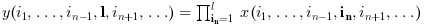Scilab-Branch-6.1-GIT
Change language to:
Français - 日本語 - Português - Русский
Scilab Help >> Elementary Functions > Matrix operations > cumprod

# cumprod

cumulative product of array elements

### Syntax

y = cumprod(x)
y = cumprod(x, orientation)
y = cumprod(x, outtype)
y = cumprod(x, orientation, outtype)

### Arguments

x

an array of reals, complex, booleans, polynomials or rational fractions.

orientation

it can be either

• a string with possible values "*", "r", "c" or "m"

• a number with positive integer value

outtype

a string with possible values "native" or "double".

y

scalar or array

### Description

For an array x, y=cumprod(x) returns in the scalar y the cumulative product of all the elements of x.

y=cumprod(x,orientation) returns in y the cumulative product of x along the dimension given by orientation:

• if orientation is equal to 1 or "r" then:or• if orientation is equal to 2 or "c" then:or• if orientation is equal to n then:• y=cumprod(x,"*") is equivalent to y=cumprod(x)

• y=cumprod(x,"m") is equivalent to y=cumprod(x,orientation) where orientation is the index of the first dimension of x that is greater than 1. This option is used for Matlab compatibility.

The outtype argument rules the way the product is done:

• For arrays of floats, of polynomials, of rational fractions, the evaluation is always done using floating points computations. The "double" or "native" options are equivalent.

• For arrays of integers,

if outtype="native" the evaluation is done using integer computations (modulo 2^b, where b is the number of bits used),

if outtype="double" the evaluation is done using floating point computations.

The default value is outtype="native".

• For arrays of booleans,

if outtype="native" the evaluation is done using boolean computations (* is replaced by &),

if outtype="double" the evaluation is done using floating point computations (%t values are replaced by 1 and %f values by 0).

The default value is outtype="double".This function applies, with identical rules to sparse matrices

### Examples

A=[1,2;3,4];
cumprod(A)
cumprod(A,1)

I=uint8([2 95 103;254 9 0])
cumprod(I) //native evaluation
cumprod(I,"double")
cumprod(I,2,"double")

s=poly(0,"s");
P=[s,%i+s;s^2,1];
cumprod(P),
cumprod(P,2)

B=[%t %t %f %f];
cumprod(B) //evaluation in float
cumprod(B,"native") //similar to and(B)